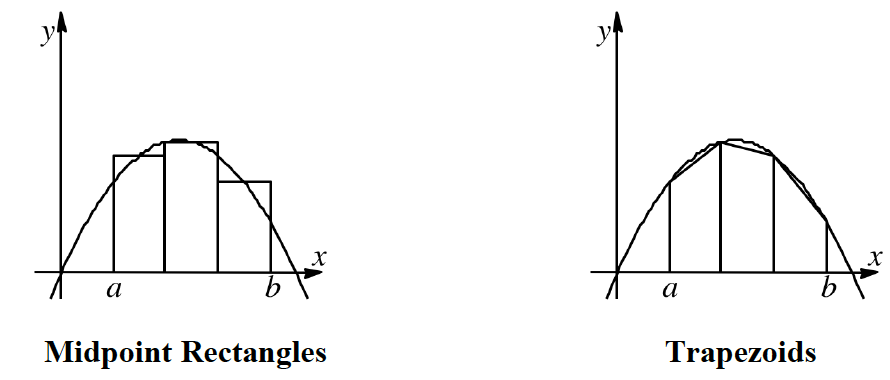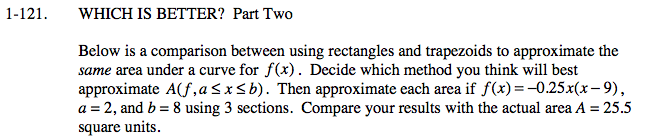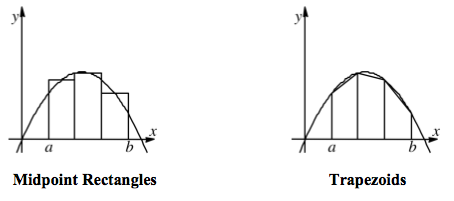### Home > CALC > Chapter 1 > Lesson 1.3.2 > Problem1-121

1-121.
1. WHICH IS BETTER? Part Two
Below is a comparison between using rectangles and trapezoids to approximate the same area under a curve for f(x). Decide which method you think will best approximate A(f, axb). Then approximate each area if f(x) = −0.25x(x − 9), a = 2, and b = 8 using 3 sections. Compare your results with the actual area A = 25.5 square units. Homework Help ✎

2.Which method comes closer to the actual curve f(x)?

Each section is 2 units wide. Use this knowledge and the equation of the function to find the areas of each section for both methods.

Which method gave you the number closest to 25.5?